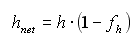# HOMER Pro 3.11Type: Intermediate Variable Units: m Symbol: hnet

One can model the friction pressure loss in the pipeline between the intake and the turbine as a loss in head. The effective head is the actual vertical drop minus this head loss. HOMER calculates the effective head (or net head) using the following equation:where: h = available head [m] fh = pipe head loss [%]

HOMER uses the effective head to calculate the power output of the hydro turbine in each time step.